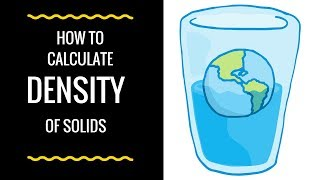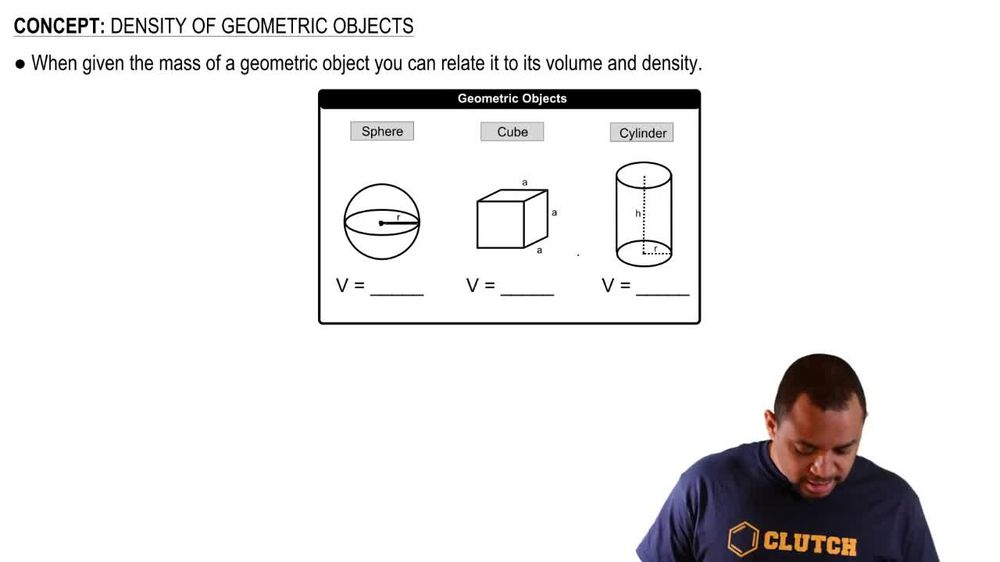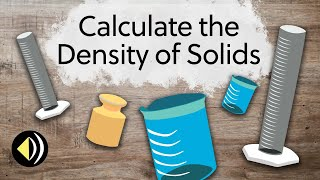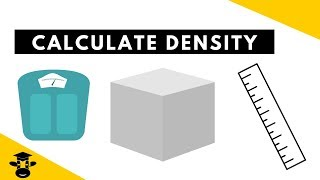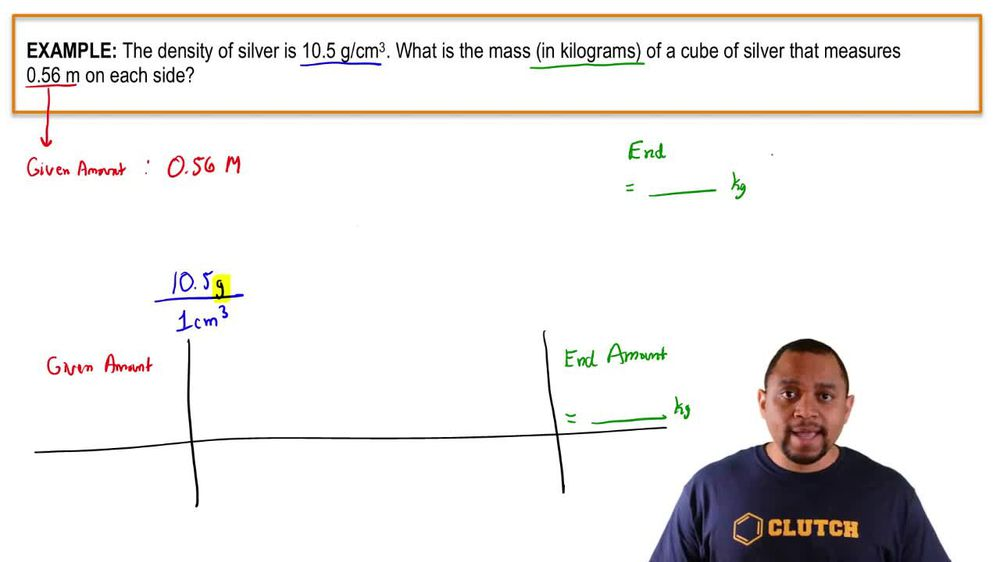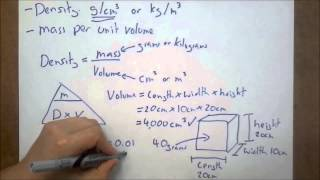Start typing, then use the up and down arrows to select an option from the list.
1. 1. Intro to General Chemistry2. Density of Geometric Objects
Problem

# What is the density of lead in g/cm3 if a rectangular bar mea-suring 0.50 cm in height, 1.55 cm in width, and 25.00 cm in length has a mass of 220.9 g?

Relevant Solution1m
Play a video:
Hey everyone, we're asked to calculate the density in grams per cubic centimeters of a rectangular tungsten bar weighing 415.5 g. With the following measurements, a height of 0.800 centimeters a width of 2.30 centimeters and a length of 11.7 centimeters. Now we've learned that our density is going to equal our mass over volume Since they've given us our mass of 415.5 g. All we need to do is find our volume and then we can find our density. As we've learned for a rectangle. The volume is going to be our length times are with times our height plugging these values in we have 11.7 cm as our length, our width as 2.30 centimeters And our height is 0.800 cm. When we calculate this out, we end up with 21.5 to a cubic cm. Now to calculate our density, We're going to take our mass of 415.5 g and divide that by our volume of 21. cubic centimeters. And this will get us to a density of 19.3 g over cubic cm, which is exactly the units that our question wanted us to give the answer in. Now I hope that made sense. And let us know if you have any questions